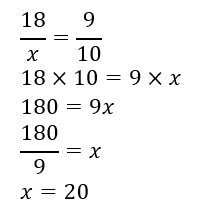# Missing Proportions Worksheets

How to Find Missing Parts of Equivalent Ratios - Ratios are mathematical expressions where two values with the same units are compared. Ratios are a quantitative relationship between two categories. When solving ratios, you will come across the concept of equivalent ratios. Ratios are said to be equivalent when each of them expresses the same relationship. To determine whether two ratios are equivalent, you can use the cross-multiplication method. Cross-multiplication is when you multiply the numerator of one ratio with the denominator of the other ratio and denominator of the first ratio with the numerator of the second ratio. If the result of both products is equal, the ratios are said to be equivalent. We can use the same cross-multiplication method to find the missing parts of an equivalent ratio. Consider the following example;This is how you find missing parts of equivalent ratios.

• ### Basic Lesson

Guides students through finding missing parts of proportions. The given ratio is proportional, find n: n:7 = 15:35 First convert fraction into simple form.

• ### Intermediate Lesson

Demonstrates how to use algebra skills to find missing proportions. The given ratio is proportional, find p: 14 to p = 7 to 9 Since they are proportional, find the ratio between given pairs.

• ### Independent Practice 1

A really great activity for allowing students to understand the concepts of missing proportions.

• ### Independent Practice 2

Students determine missing proportions in 20 assorted problems. The answers can be found below.

• ### Homework Worksheet

Students are provided with 12 problems to achieve the concepts of missing proportions.

• ### Skill Quiz

This tests the students ability to determine missing parts of proportions.

• ### Homework and Quiz Answer Key

Answers for the homework and quiz.

Answers for the lesson and practice sheets.

• ### Basic Lesson

Guides students through the algebra skills necessary to solve proportions with a missing variable.

• ### Intermediate Lesson

Demonstrates how to reorder terms within a proportion problem.

• ### Independent Practice 1

A really great activity for allowing students to understand the concepts of solving proportions.

• ### Independent Practice 2

Students Rewrite Ratios in 20 assorted problems. The answers can be found below.

• ### Homework Worksheet

Students are provided with 12 problems that are great to take home and review.

• ### Skill Quiz

This tests the students ability on all aspects of this unit.

• ### Homework and Quiz Answer Key

Answers for the homework and quiz.